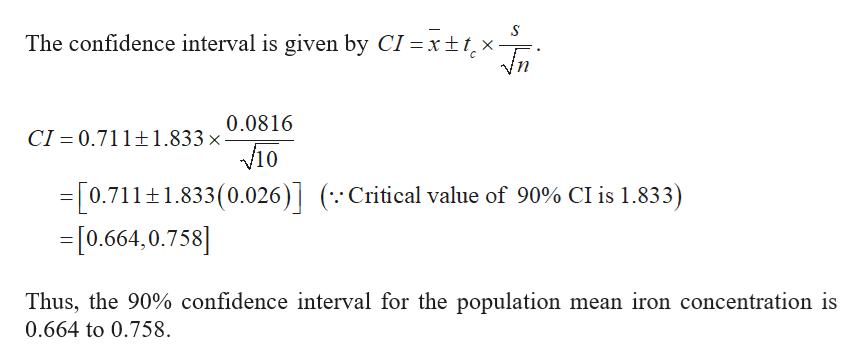# A physicist examines 1010 water samples for iron concentration. The mean iron concentration for the sample data is 0.7110.711 cc/cubic meter with a standard deviation of 0.08160.0816. Determine the 90%90% confidence interval for the population mean iron concentration. Assume the population is approximately normal.Find the critical value that should be used in constructing the confidence interval. Round your answer to three decimal places.

Question
23 views

A physicist examines 1010 water samples for iron concentration. The mean iron concentration for the sample data is 0.7110.711 cc/cubic meter with a standard deviation of 0.08160.0816. Determine the 90%90% confidence interval for the population mean iron concentration. Assume the population is approximately normal.

Find the critical value that should be used in constructing the confidence interval. Round your answer to three decimal places.

check_circle

Step 1

It is given that the standard deviation is 0.0816, mean is 0.711 and the sample size is 10.

The 90% of the confidence interval for the populatio...help_outlineImage TranscriptioncloseThe confidence interval is given by CI =xt tx 0.0816 CI 0.711+1.833 x 10 =[0.7111.833(0.026)(Critical value of 90% CI is 1.833) -[0.664,0.758] Thus, the 90% confidence interval for the population mean iron concentration is 0.664 to 0.758. fullscreen

### Want to see the full answer?

See Solution

#### Want to see this answer and more?

Solutions are written by subject experts who are available 24/7. Questions are typically answered within 1 hour.*

See Solution
*Response times may vary by subject and question.
Tagged in